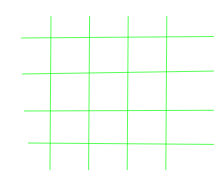# Ways to place 4 items in n^2 positions such that no row/column contains more than one

Given an integer N where 4 ≤ N ≤ 100. There are N lines vertically and N lines horizontally. So, There are N2 intersections. The task is find the number of ways to place 4 items in these N2 positions such that each row and column contain not more than one item.

Examples:

Input: N = 4Output: 24

Input: N = 5
Output: 600

## Recommended: Please try your approach on {IDE} first, before moving on to the solution.

Approach: The number of ways to choose 4 horizontal lines that will have items from n is nC4. There are n ways to place an item on the first of these lines. Given the place of the first item, there are n – 1 ways to place an item on the second of these lines because one of the vertical lines is already taken. Given the places of the first and second items, there are n – 2 ways to place an item on the third line and the same way n – 3 for the fourth item. The total number of ways to place items on selected 4 horizontal paths is n * (n – 1) * (n – 2) * (n – 3). So the result is nC4 * n * (n – 1) * (n – 2) * (n – 3).

Below is the implementation of the above approach:

## C++

 `// C++ implementation of the approach ` `#include ` `using` `namespace` `std; ` ` `  `// Function to return the number of ways ` `// to place 4 items in n^2 positions ` `long` `long` `NumberofWays(``int` `n) ` `{ ` `    ``long` `long` `x = (1LL * (n) * (n - 1) * (n - 2) * (n - 3)) ` `                  ``/ (4 * 3 * 2 * 1); ` `    ``long` `long` `y = (1LL * (n) * (n - 1) * (n - 2) * (n - 3)); ` ` `  `    ``return` `(1LL * x * y); ` `} ` ` `  `// Driver code ` `int` `main() ` `{ ` `    ``int` `n = 4; ` `    ``cout << NumberofWays(n); ` ` `  `    ``return` `0; ` `} `

## Java

 `// Java implementation of the approach ` `class` `GFG ` `{ ` ` `  `// Function to return the number of ways  ` `// to place 4 items in n^2 positions  ` `static` `long` `NumberofWays(``int` `n)  ` `{  ` `    ``long` `x = (1l * (n) * (n - ``1``) * (n - ``2``) * (n - ``3``))  ` `                ``/ (``4` `* ``3` `* ``2` `* ``1``);  ` `    ``long` `y = (1l * (n) * (n - ``1``) * (n - ``2``) * (n - ``3``));  ` ` `  `    ``return` `(1l * x * y);  ` `}  ` ` `  `// Driver code  ` `public` `static` `void` `main(String args[]) ` `{  ` `    ``int` `n = ``4``;  ` `    ``System.out.println( NumberofWays(n));  ` `}  ` `} ` ` `  `// This code is contributed by Arnab Kundu `

## Python3

 `# python implementation of the approach  ` ` `  `# Function to return the number of ways  ` `# to place 4 items in n^2 positions  ` `def` `NumbersofWays(n): ` `    ``x ``=` `(n ``*` `(n ``-` `1``) ``*` `(n ``-` `2``) ``*` `(n ``-` `3``)) ``/``/` `(``4` `*` `3` `*` `2` `*` `1``) ` `    ``y ``=` `n ``*` `(n ``-` `1``) ``*` `(n ``-` `2``) ``*` `(n ``-` `3``) ` ` `  `    ``return` `x ``*` `y ` ` `  `# Driver code ` `n ``=` `4` `print``(NumbersofWays(n)) ` ` `  `# This code is contributed by Shrikant13 `

## C#

 `// C# implementation of the approach  ` `using` `System; ` ` `  `class` `GFG ` `{ ` ` `  `// Function to return the number of ways  ` `// to place 4 items in n^2 positions  ` `public` `static` `long` `NumberofWays(``int` `n) ` `{ ` `    ``long` `x = (1l * (n) * (n - 1) * (n - 2) *  ` `               ``(n - 3)) / (4 * 3 * 2 * 1); ` `    ``long` `y = (1l * (n) * (n - 1) * (n - 2) *  ` `                ``(n - 3)); ` ` `  `    ``return` `(1l * x * y); ` `} ` ` `  `// Driver code  ` `public` `static` `void` `Main(``string``[] args) ` `{ ` `    ``int` `n = 4; ` `    ``Console.WriteLine(NumberofWays(n)); ` `} ` `} ` ` `  `// This code is contributed by Shrikant13 `

## PHP

 ` `

Output:

```24
```My Personal Notes arrow_drop_upCheck out this Author's contributed articles.

If you like GeeksforGeeks and would like to contribute, you can also write an article using contribute.geeksforgeeks.org or mail your article to contribute@geeksforgeeks.org. See your article appearing on the GeeksforGeeks main page and help other Geeks.

Please Improve this article if you find anything incorrect by clicking on the "Improve Article" button below.